Most Affordable JEE | NEET | 8,9,10 Preparation by Kota's Top IITian Doctor Faculties

# Structure of Atom Class 11 Questions and Answers-Important for ExamsGet Structure of Atom important questions and answers for class 11 Chemistry exams. View the Important Question bank for Class 11 & 12 Chemistry developed by expert faculties from Kota. These important questions will play significant role in clearing concepts of Chemistry. This question bank is designed, keeping NCERT in mind and the questions are updated with respect to upcoming Board exams. You will get here all the important questions for class 11 chemistry chapter. Learn all the concepts of Structure class 11 chemistry of Atom Chapter through these questions and answers

Click Here for Detailed Chapter-wise Notes of Chemistry for Class 11th, JEE & NEET.  You can access free study material for all three subject’s Physics, Chemistry and Mathematics. Click Here for Detailed Notes of any chapter.  eSaral provides you complete edge to prepare for Board and Competitive Exams like JEE, NEET, BITSAT, etc. We have transformed classroom in such a way that a student can study anytime anywhere. With the help of AI we have made the learning Personalized, adaptive and accessible for each and every one. Visit eSaral Website to download or view free study material for JEE & NEET. Also get to know about the strategies to Crack Exam in limited time period.

Q. How many protons and neutrons are present in the following nuclei?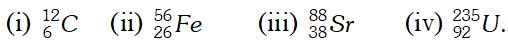[NCERT]
Ans. (i) 6, 6 (ii) 26, 30 (iii) 38, 50 (iv) 92, 143.
Q. The diameter of zinc atom is 2.6 A. Calculate (i) the radius of zinc atom in pm (ii) number of atoms present in a length of 1.6 cm if the zinc atoms are arranged side by side lengthwise. [NCERT]
Ans.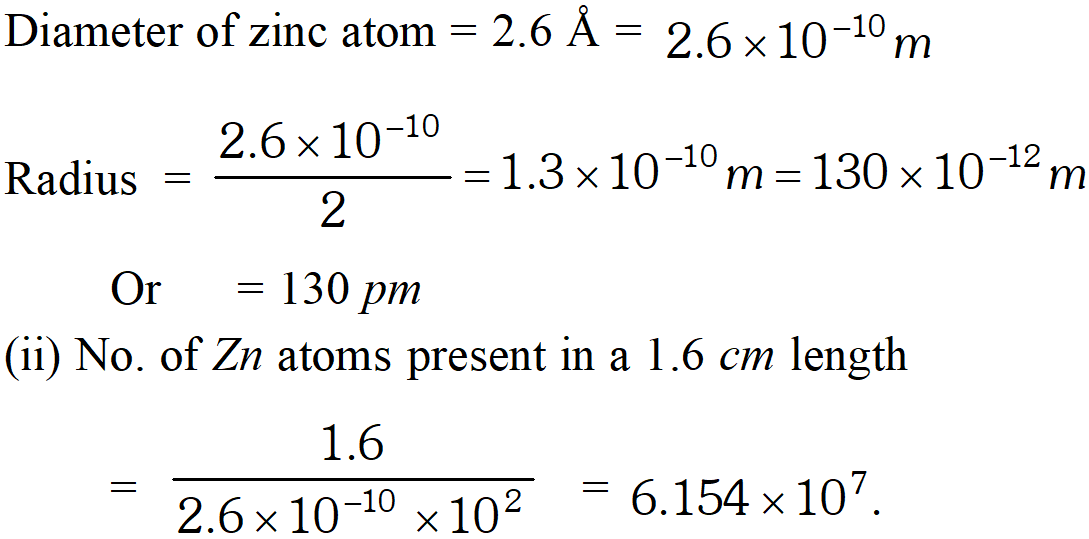Q. A certain particle carries $2.5 \times 10^{-16} \mathrm{C}$ of static electric charge. Calculate the number of electrons present in it. [NCERT]
Ans. Charge carried by one electron $=1.6022 \times 10^{-19} \mathrm{C}$ $\therefore \quad$ Electrons present in particle carrying $2.5 \times 10^{-16} \mathrm{C}$ charge $=\frac{2.5 \times 10^{-16}}{-1.6022 \times 10^{-19}}=1560$
Q. Among the following pairs of orbitals which orbital will experience the larger effective nuclear charge ? (a) $2 s$ and $3 s,$ (b) $4 d$ and $4 f,$ (c) [NCERT]
Ans. (i) $2 s$ is closer to the nucleus than 3 s. Hence 2 swill experience larger effective nuclear charge. (ii) $4 d$ (iii) $3 p$ (for same reasons).
Q. The unpaired electrons in $A l$ and $S i$ are present in $3 p$ orbital. Which electron will experience more effective charge from the nucleus? [NCERT]
Ans. Silicon has greater nuclear charge $(+14)$ than aluminium $(+13) .$ Hence, the unpaired $3 p$ electron in case of silicon will experience more effective nuclear charge.
Q. Arrange the following type of radiations in increasing order of frequency: (a) radiation from microwave oven, (b) amber light. from traffic signal, (c) radiation from FMradio, (d) cosmic rays from outer space and (e) $X$ -rays. [NCERT]
Ans. The increasing order of frequency is : Radiation from FM radio < radiation from microwave oven < amber light from traffic signal < X-rays < cosmic rays from outer space.
Q. The energy associated with the first orbit of hydrogen atom is $-2.17 \times 10^{-18} \mathrm{J} /$ atom. What is the energy associated with the fifth orbit? [NCERT]
Ans. $E_{n}=\frac{E_{1}}{n^{2}} ; E_{5}=\frac{-2.17 \times 10^{-18}}{(5)^{2}} \mathrm{J} /$ atom $=-8.68 \times 10^{-20} \mathrm{J} /$ atom.
Q. Nitrogen laser produces a radiation at a wavelength of 337.1 nm. If the number of photons emitted is $5.6 \times 10^{24}$, calculate the power of this laser. [NCERT]
Ans. $E=N h v=N h \frac{c}{\lambda} \quad\left(\because v=\frac{c}{\lambda}\right)$ $=\frac{\left(5.6 \times 10^{24}\right)\left(6.626 \times 10^{-34} \mathrm{J} \mathrm{s}\right)\left(3.0 \times 10^{8} \mathrm{ms}^{-1}\right)}{\left(337.1 \times 10^{-9} \mathrm{m}\right)}$ $=3.3 \times 10^{6} J$
Q. What is meant by quantization of energy ?
Ans. Quantization of energy means the energy of energy levels can have some specific values of energy and not all the values.
Q. Write the mathematical expression by which the energies of various stationary states in the hydrogen atom can be calculated.
Ans. $\mathrm{E}_{\mathrm{n}}=-\frac{1312 \mathrm{kJ} \mathrm{mol}^{-1}}{\mathrm{n}^{2}}$
Q. How many subshells associated with $n=4 ?$ How many electrons will be present in the subshell having $m_{s}$ value of $-1 / 2$ for $n=4 ?$
Ans. (a) Subshells in $n=4$ are $4 s(l=0), 4 p(l=1), 4 d(l=2), 4 f(l=3)$ The number of electrons having $m_{s}=-\frac{1}{2}$ in $n=4$ will be 16
Q. Explain the meaning of the symbol $4 d^{6} ?$ [NCERT]
Ans. It means that 4d sub-shell has 6 electrons. 4 represents fourth energy shell and d is a sub-shell and 6 electrons are present in d orbitals of sub-shell.
Q. How does Heisenberg's Uncertainty principle support concept of orbital?
Ans. According to Heisenberg’s Uncertainty principle ‘the exact position of electron cannot be determined, i.e., the exact path of electron cannot be determined but we can determine the region or space where electron spends most of its time, and this region or space is called orbital.
Q. Explain why the uncertainty principle is significant only for the motion of subatomic particles but is negligible for the macroscopic objects.
Ans. The energy of photon is sufficient to disturb a subatomic particle so that there is uncertainty in the measurement of position and momentum of the subatomic particle. However, the energy is insufficient to disturb a macroscopic object.
Q. Can we apply Heisenberg’s uncertainty principle to a stationary electron ? Why or why not?
Ans. No, because, velocity = 0 and position can be measured accurately.
Q. Which of the four quantum numbers $\left(n, l, m_{l}, m_{s}\right)$ determine: (i) the energy of an electron in a hydrogen atom and multi electron atoms (ii) the size of an orbital (iii) the shape of an orbital (iv) the orientation of an orbital in space ? [NCERT]
Ans. (i) n (ii) n (iii) l (iv) $m_{l}$
Q. What physical meaning is attributed to the square of the absolute value of wave function $\left|\psi^{2}\right| ?$ [NCERT]
Ans. It measures the electron probability density at a point in an atom.
Q. How many electrons in an atom may have the following quantum number ? (i) $n=4, m_{s}=+1 / 2$ (ii) $n=3, l=0$ [NCERT]
Ans. (i) $n=4, m_{\mathrm{s}}=+1 / 2=16$ electrons (ii) $n=3, l=0=2$
Q. For each of the following pair of hydrogen orbitals, indicate which is higher in energy : (i) $1 s, 2 s$ (ii) $2 p, 3 p$ (iii) $3 d_{x y}, 3 d_{y z}$ (iv) $3 s, 3 d$ (v) $4 f, 5 s$ [NCERT]
Ans. (i) $2 s$ (ii) $3 p$ (iii) both have same energy (iv) both have same energy (v) $5 \mathrm{s}$
Q. The unpaired electrons in $A l$ and $S i$ are present in $3 p$ orbital. Which electrons will experience more effective nuclear charge from the nucleus? [NCERT]
Ans. Si
Q. An electron is in one of the $3 d$ orbitals. Give the possible values of $n, l,$ and $m_{l}$ for this electron. [NCERT]
Ans. $\mathrm{n}=3,1=2, \mathrm{m}_{1}=+2,+1,0,-1,-2$
Q. List the quantum numbers ( $l$ and $m_{l}$ ) for electrons in 3d orbital. [NCERT]
Ans. $1=2,$ and $\mathrm{m}_{1}=+2,+1,0,-1,-2$
Q. Give the number of electrons in the species $H_{2}^{+}, H_{2}$ and $O_{2}^{+}$
Ans. Number of electrons : $H_{2}^{+}=1 ; H_{2}=2 ; \quad O_{2}^{+}=15$
Q. What atoms are indicated by the following configurations: (i) $[\mathrm{He}] 2 \mathrm{s}^{1}$ (ii) $[\mathrm{Ne}] 3 \mathrm{s}^{2} 3 \mathrm{p}^{3} \quad$ (iii) $[\mathrm{Ar}] 4 \mathrm{s}^{2} 3 \mathrm{d}^{1}$ [NCERT]
Ans. (i) $L i$ (ii) $P \quad$ (iii) $S c$
Q. $2 \times 10^{8}$ atoms of carbon are arranged side by side. Calculate the radius of carbon atoms if length of this arrangement is $2.4 \mathrm{cm}$
Ans. An atom will cover length equal to its diameter Diameter of carbon atom $=\frac{2.4}{2 \times 10^{8}}$ $=1.2 \times 10^{-8} \mathrm{cm}=1.2 \times 10^{-10} \mathrm{m}$ Radius of carbon atom $=\frac{1.2 \times 10^{-10}}{2}=0.6 \times 10^{-10} \mathrm{m}$ $=0.06 \times 10^{-9} \mathrm{m}=0.06 \mathrm{nm}$
Q. The wavelength range of the visible spectrum extends from = violet $(400 \mathrm{nm})$ to red $(750 \mathrm{nm}) .$ Express their wave-lengths in frequencies $(H z) .\left(1 n m=10^{-9} m\right)$ [NCERT]
Ans. For violet light $v=7.50 \times 10^{14} \mathrm{Hz}$ For red light $v=4.00 \times 10^{14} \mathrm{Hz}$
Q. An ion with mass number 56 contains 3 units of positive charge and 30.4% more neutrons than electrons. Assign the symbol for the ion. [NCERT]
Ans. since the ion carries 3 units of positive charge, it will have 3 electrons less than the number of protons. Let number of electrons $=x$ No. of protons $=x+3$ No. of neutrons $=x+\frac{x \times 30.4}{100}$ $=x+0.304 x=1.304 x$ Now, No. of protons $+$ No. of neutrons $=56$ $x+3+1.304 x=56$ $2.304 x=53$ $x=\frac{53}{2.304}=23$ No. of electrons $=23,$ No. of protons $=23+3=26$ No. of neutrons $=56-26=30$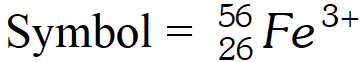Q. An element with mass number 81 contains 31.7% more neutrons as compared to protons. Assign the atomic symbol. [NCERT]
Ans. We know, Mass number $=$ No. of protons $+$ No. of neutrons $=81$ i.e., $p+n=81$ Let number of protons $=x$ Number of neutrons $=x+\frac{x \times 31.7}{100}=1.317 x$ $\therefore \quad x+1.317 x=81$ $x=\frac{81}{2.317}=34.96=35$ Symbol $=\begin{array}{l}{81} \\ {35}\end{array} B r$
Q. An ion with mass number 37 possesses one unit of negative charge. If the ion contains 11.1% more neutrons than the electrons, find the symbol of the ion. [NCERT]
Ans. Since the ion carries one unit of negative charge, it means that in the ion the number of electrons is one more than the number of protons. Total number of electrons and neutrons in the ion = 37 + 1 = 38 Let the number of electrons in the ion be = x Number of neutrons in the $=\frac{x \times 111.1}{100}=1.111 x$ $=x+1.111 x=2.111 x$ $2.111 x=38$ $x=\frac{38}{2.111}=18$ $\therefore$ Number of electrons in the ion $=18$ Number of protons in the ion $=18-1=17$ Thus, atomic number of the elements is 17 which corresponds to chlorine. $\therefore$ Symbol of the ion is $_{17}^{37} \mathrm{Cl}^{-}$
Q. The threshold frequency $v_{o}$ for a metal is $7.0 \times 10^{14} \mathrm{s}^{-1}$ Calculate the kinetic energy of an electron emitted when radiation of $v=1.0 \times 10^{15} \mathrm{s}^{-1}$ hits the metal. [NCERT]
Ans. $K . E .=\frac{1}{2} m v^{2}=\frac{1}{2} m_{e} v^{2}=h\left(v-v_{0}\right)$ $=\left(6.626 \times 10^{-34} \mathrm{Js}\right)\left(1.0 \times 10^{15} \mathrm{s}^{-1}-7.0 \times 10^{14} \mathrm{s}^{-1}\right)$ $=\left(6.626 \times 10^{-34} \mathrm{Js}\right)\left(10 \times 10^{14} \mathrm{s}^{-1}-7.0 \times 10^{14} \mathrm{s}^{-1}\right)$ $=\left(6.626 \times 10^{-34} \mathrm{Js}\right)\left(3.0 \times 10^{14} \mathrm{s}^{-1}\right)=1.988 \times 10^{-19} \mathrm{J}$
Q. The Vividh Bharti station of All India Radio, Delhi broadcasts on a frequency of 1,368 kHz. Calculate the wavelength of the electromagnetic radiation emitted by the transmitter. Which part of the electromagnetic spectrum does it belong ? [NCERT]
Ans. The wavelength, $\lambda,$ is equal to $c / v$ where $^{t} c^{\prime}$ is the speed of electromagnetic radiation in vacuum and ' $v^{\prime}$ is the frequency. Substituting the given values, we have, $\lambda=\frac{3.00 \times 10^{8} \mathrm{ms}^{-1}}{1368 \mathrm{kHz}}$ $=\frac{3.00 \times 10^{8} \mathrm{ms}^{-1}}{1368 \times 10^{3} \mathrm{s}^{-1}}=219.3 \mathrm{m}$ This is a characteristic radiowave wavelength.
Q. Emission transitions in the Paschen series end at orbit $n=3$ and start from an orbit $n$ and can be represented as $v=3.29 \times 10^{15}(\mathrm{Hz})\left(\frac{1}{3^{2}}-\frac{1}{n^{2}}\right)$ Calculate the value of $n$ if the transition is obtained at $1285 \mathrm{nm} .$ Find the region of spectrum. [NCERT]
Ans. $v=3.29 \times 10^{15}\left(\frac{1}{3^{2}}-\frac{1}{n^{2}}\right) s^{-1}$ $\lambda=1285 \mathrm{nm}$ $\therefore \quad v=\frac{c}{\lambda}=\frac{3.0 \times 10^{8} \mathrm{ms}^{-1}}{1285 \times 10^{-9} \mathrm{m}}$ $=2.33 \times 10^{14} s^{-1}$ $2.33 \times 10^{14}=3.29 \times 10^{15}\left(\frac{1}{9}-\frac{1}{n^{2}}\right)$ or $\quad \frac{1}{9}-\frac{1}{n^{2}}=\frac{2.33 \times 10^{14}}{3.29 \times 10^{15}}=0.0708$ $|-\frac{1}{n^{2}}=0.0708-\frac{1}{9}=-0.0403$ $\frac{1}{n^{2}}=0.0403$ or $n^{2}=24.82$ $\therefore \quad n=5$
Q. Electrons are emitted with zero velocity from a metal surface. when it is exposed to radiation of wavelength $6,800$ A. Calculate threshold frequency $\left(v_{0}\right)$ and work function $\left(W_{0}\right)$ of the metal.
Ans. $h v=h \theta_{\circ}+\frac{1}{2} m v^{2} \quad$ since $v=0$ $h v=h v_{o}+0$ $h v=h v_{0} \Rightarrow v=v_{0}$ $v=\frac{c}{\lambda} \Rightarrow v=\frac{3 \times 10^{8} \mathrm{ms}^{-1}}{6800 \times 10^{-10} \mathrm{m}}=\frac{300}{86} \times 10^{14}=4.41 \times 10^{14} \mathrm{s}^{-1}$ $v=v_{0}=4.41 \times 10^{14} \mathrm{s}^{-1}$ $W_{o}=h v_{o}=6.626 \times 10^{-34} \mathrm{Js} \times 4.41 \times 10^{14} \mathrm{s}^{-1}=2.91 \times 10^{-19} \mathrm{J}$
Q. Calculate the energy associated with the first orbit of $H e^{+}$ What is the radius of this orbit? [NCERT]
Ans. Energy of electron in nth orbit is $E_{n}=-\frac{13.595}{n^{2}} Z^{2} e V$ atom $^{-1}$ For first orbit of $H e^{+}, Z=2, n=1$ $E_{1}=-\frac{13.595 \times(2)^{2}}{1^{2}}=-54.380 e V$ Radius of nth orbit is, $r_{n}=\frac{0.529 n^{2}}{Z} A$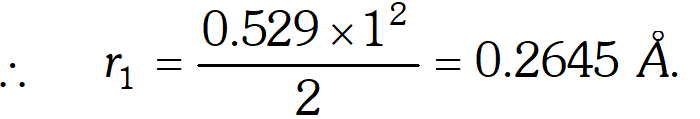Q. Calculate the wave number for the longest wavelength transition in Balmer series of atomic hydrogen. [NCERT]
Ans. The longest wavelength corresponds to minimum energy $(\Delta E)$ transition. For Balmer series this transition is from $n_{2}=3$ to $n_{1}=2$ $v=109677\left[\frac{1}{n_{1}^{2}}-\frac{1}{n_{2}^{2}}\right] \mathrm{cm}^{-1}$ $=109677\left[\frac{1}{(2)^{2}}-\frac{1}{(3)^{2}}\right] \mathrm{cm}^{-1}$ $=15233 \mathrm{cm}^{-1}=1.5233 \times 10^{4} \mathrm{cm}^{-1}$ or $\quad=1.5233 \times 10^{6} \mathrm{m}^{-1}$
Q. Show that the circumference of the Bohr orbit for hydrogen atom is an integral multiple of the de Broglie wavelength associated with the electron moving around the orbit. [NCERT]
Ans. According to Bohr postulate of angular momentum,. $m v r=n \frac{h}{2 \pi} \quad \text { or } \quad 2 \pi r=n \frac{h}{m v} \quad \ldots .(\mathrm{i})$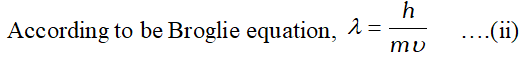Substituting this value in equation (i), we get $2 \pi r=n \lambda$ Thus, the circumference $(2 \pi r)$ of the Bohr orbit for hydrogen atoms is an integral multiple of de-Broglie wavelength.
Q. Calculate de-Broglie wavelength of an electron (mass $=9.1 \times 10^{-31} \mathrm{kg}$ ) moving at $1 \%$ speed of light. $\left(h=6.63 \times 10^{-34} \mathrm{kg} \mathrm{m}^{2} \mathrm{s}^{-1}\right)$ [NCERT]
Ans. We know that $\lambda=\frac{h}{m v}$ $m=9.1 \times 10^{-31} \mathrm{kg}, h=6.63 \times 10^{-34} \mathrm{kg} \mathrm{m}^{2} \mathrm{s}^{-1}$ $v=1 \%$ of speed of light $=\frac{1 \times 3.0 \times 10^{8}}{100} m s^{-1}$ $=3.0 \times 10^{6} \mathrm{ms}^{-1}\left(\because \text { speed of light }=3.0 \times 10^{8} \mathrm{ms}^{-1}\right)$
Q. The mass of an electron is $9.1 \times 10^{-31} \mathrm{kg} .$ If its K.E. is $3.0 \times 10^{-25} \mathrm{J},$ calculate its wavelength. [NCERT]
Ans. $K . E .=\frac{1}{2} m v^{2}$ $\therefore v=\sqrt{\frac{2 K \cdot E}{m}}=\sqrt{\frac{2 \times 3.0 \times 10^{-25} \mathrm{J}}{9.1 \times 10^{-31} \mathrm{kg}}=812} \mathrm{ms}^{-1}$ $\left(1, J=1 k g m^{2} s^{-2}\right)$ By de-Broglie equation, $\lambda=\frac{h}{m v}=\frac{6.626 \times 10^{-34} \mathrm{Js}}{\left(9.1 \times 10^{-31} \mathrm{kg}\right)\left(812 \mathrm{ms}^{-1}\right)}$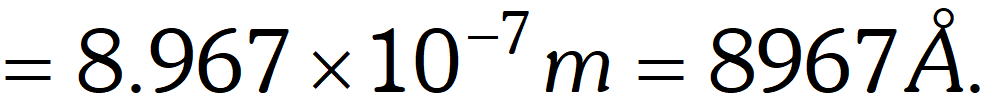Q. (i) How many sub-shells are associated with $n=4$ ? (ii) How many electrons will be present in the sub-shells having $m_{s}$ value of $-1 / 2$ for $n=4 ?$
Ans. (i) $n=4, l=0,1,2,3,(4 \text { subshells, viz, } s, p, d \text { and } f)$ (ii) No. of orbitals in 4 th shell $=n^{2}=4^{2}=16$ Each orbital has one electron with $m_{s}=-1 / 2 .$ Hence, there will be 16 electrons with $m_{s}=-1 / 2$
Q. Calculate the uncertainty in the velocity of a wagon of mass 2000 kg whose position is known to an accuracy of \pm10 $\mathrm{m}$ [NCERT]
Ans. Mass of wagon $=2000 \mathrm{kg}$ Uncertainty in position, $\Delta x=\pm 10 \mathrm{m}$ According to Heisenberg uncertainty principle $\Delta x \times \Delta p=\frac{h}{4 \pi}$ or $\Delta x \times \Delta v=\frac{h}{4 \pi m}$ or $\quad \Delta v=\frac{h}{4 \pi m \Delta x}$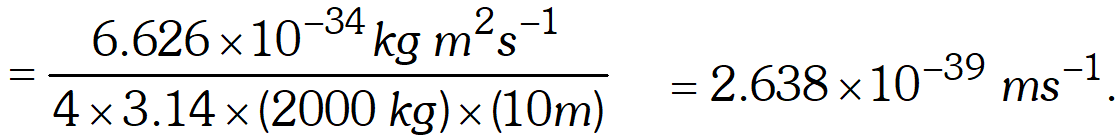Q. If the position of the electron is measured within an accuracy of $\pm 0.002 n m,$ calculate the uncertainty in the momentum of the electron. Suppose the momentum of the electron is $h / 4 \pi \times 0.05 n m,$ is there any problem in defining this value. [NCERT]
Ans. $\Delta x=0.002 n m=2 \times 10^{-3} n m=2 \times 10^{-12} m$ $\Delta x \times \Delta p=\frac{h}{4 \pi}$ $\therefore \quad \Delta p=\frac{h}{4 \pi \Delta x}=\frac{6.626 \times 10^{-34} \mathrm{kg} m^{2} s^{-1}}{4 \times 3.14 \times\left(2 \times 10^{-12} \mathrm{m}\right)}$ $=2.638 \times 10^{-23} \mathrm{kg} \mathrm{ms}^{-1}$ Actual momentum $=\frac{h}{4 \pi \times 0.05 \mathrm{nm}}=\frac{h}{4 \pi \times 5 \times 10^{-11} \mathrm{m}}$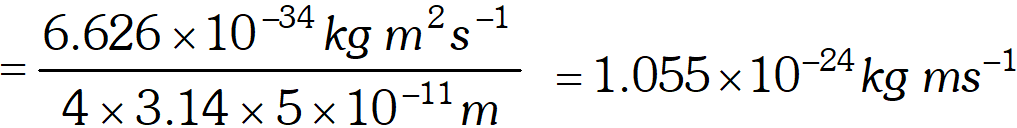It cannot be defined as the actual magnitude of the momentum is smaller than the uncertainty.
Q. Using the s, p, d notations, describe the orbital with the following quantum numbers :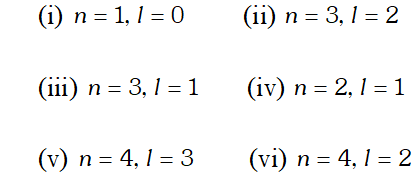[NCERT]
Ans.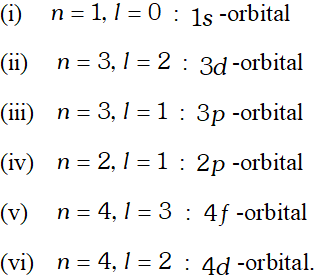Q. How many sub-shells are associated with $n=4$ ? [NCERT]
Ans. For $n=4, l$ can have values $0,1,2,3 .$ Thus, there are four sub-shells in $n=4$ energy level. These four sub-shells are $4 s, 4 p, 4 d$ and $4 f$
Q. Indicate the number of unpaired electron in (i) $P(\text { ii })$ Si ( iii) $C r(\text { iv })$ Fe, and (v) $K r$ [NCERT]
Ans. i) $\quad_{15} P=1 s^{2} 2 s^{2} 2 p^{6} 3 s^{2} 3 p_{x}^{1} 3 p_{y}^{1} 3 p_{z}^{1}$ No. of unpaired electron $=3$ (ii) $\quad_{14} S i=1 s^{2} 2 s^{2} 2 p^{6} 3 s^{2} 3 p_{x}^{1} 3 p_{y}^{1}$ No. of unpaired electron $=2$ (iii) $\quad_{24} C r=1 s^{2} 2 s^{2} 2 p^{6} 3 s^{2} 3 p^{6} 3 d^{5} 4 s^{1}$ No. of unpaired electrons $=6$ (iv) $\quad 26 F e=1 s^{2} 2 s^{2} 2 p^{6} 3 s^{2} 3 p^{6} 3 d^{6} 4 s^{2}$ No. of unpaired electrons $=4(\text { in } 3 d)$ (v) $\quad 36 K r=$ Noble gas. All orbitals are filled. Unpaired electron $=0$
Q. An atom of an element contains 29 electrons and 35 neutrons. Deduce (i) the number of protons and (ii) the electronic configuration of the element. [NCERT]
Ans. No. of protons $=$ Number of electrons $=29$ Electronic configuration $=1 s^{2} 2 s^{2} 2 p^{6} 3 s^{2} 3 p^{6} 4 s^{1} 3 d^{10}$
Q. Consider the following electronic configurations : (i) $1 s^{2} 2 s^{1}$ (ii) $1 s^{2} 3 s^{1}$ (a) Name the element corresponding to (i) (b) Does (ii) correspond to the same or different element? (c) How can (ii) be obtained from (i) ? (d) Is it easier to remove one electron from (ii) or (i) ? Explain.
Ans. (a) The element corresponding to ( i ) is Lithium (Li). (b) This electronic configuration represents the same element in the excited state. (c) By supplying energy to the element when the electron jumps from the lower energy $2 s$ -orbital to the higher energy $3 s$ orbital. (d) It is easier to remove an electron from (ii) than from (i) since in the former case the electron is present in a $3 s$ -orbital which is away from the nucleus and hence is less strongly attracted by the nucleus than an electron in the $2 s$ -orbital.
Q. What atoms are indicated by the following configuration? Are they in the ground state or excited state ? (i) $1 s^{2} 2 s^{2} 2 p_{x}^{2} 2 p_{y}^{2} 2 p_{z}^{1}$ (ii) $1 s^{2} 2 s^{1} 2 p_{x}^{1} 2 p_{y}^{1} 2 p_{z}^{1}$ (iii) $1 s^{2} 2 s^{2} 2 p^{6} 3 s^{2} 3 p_{x}^{1} 3 p_{y}^{1}$ (iv) $1 s^{2} 2 s^{2} 2 p^{6} 3 s^{1} 3 p_{x}^{1} 3 p_{y}^{1} 3 p_{z}^{1} 3 d^{1}$ (v) $[A r] 3 d^{5} 4 s^{2}$
Ans. (i) $1 s^{2} 2 s^{2} 2 p_{x}^{2} 2 p_{y}^{2} 2 p_{z}^{1} .$ Atomic number is 9 and it is configuration of fluorine in ground state. (ii) $1 s^{2} 2 s^{1} 2 p_{x}^{1} 2 p_{y}^{1} 2 p_{z}^{1} .$ Atomic number is 6 and it is configuration of carbon in excited state. (iii) $1 s^{2} 2 s^{2} 2 p^{6} 3 s^{2} 3 p_{x}^{1} 3 p_{y}^{1} .$ Atomic number is 14 and it is configuration of silicon in ground state. (iv) $1 s^{2} 2 s^{2} 2 p^{6} 3 s^{1} 3 p_{x}^{1} 3 p_{y}^{1} 3 p_{z}^{1} 3 d^{1} .$ Atomic number is 15 and it is configuration of phosphorus in excited state. (v) $[A r] 3 d^{5} 4 s^{2} .$ Atomic number is 25 and it is configuration of manganese in ground state.
Q. An atom has 2 electrons in the first $(K)$ shell, 8 electrons in the second $(L)$ shell and 2 electrons in the third $(M)$ shell. Give its electronic configuration and find out the following: (i) Atomic number (ii) Total number of principal quantum numbers (iii) Total number of sub-levels (iv) Total number of $s$ -orbitals (v) Total number of $p$ -electrons.
Ans. The electronic configuration of the atom is : $1 s^{2} 2 s^{2} 2 p^{6} 3 s^{2}$ (i) Atomic number $=2+2+6+2=12$ (ii) Number of principal quantum numbers $=3$ (iii) Number of sub-levels $=4(1 s, 2 s, 2 p, 3 s)$ (iv) Number of $s$ -orbitals $=3(1 s, 2 s, 3 s)$ (v) Total number of $p$ -electrons $=6$
Q. Account for the following: (i) The expected electronic configuration of copper is $[A r] 3 d^{9} 4 s^{2}$ but actually it is $[A r] 3 d^{10} 4 s^{1}$ (ii) In building up of atoms, the filling of $4 s$ orbitals occurs before $3 d-$ orbitals.
Ans. (i) It is because half filled and completely filled orbitals are more stable. (ii) $4 s$ orbital has lower energy than $3 d$ orbital, therefore, it is filled before $3 d$ orbital.
Q. (i) Calculate the total number of electrons present in 1 mol of methane. (ii) Find (a) the total number and (b) total mass of neutrons in $7 m g$ of $^{14} C$. (Assume mass of a neutron $\left.=1.675 \times 10^{-27} \mathrm{kg}\right)$ (iii) Find (a) total number and (b) total mass of protons in $34 m g$ of $N H_{3}$ at STP. Will the answer change if the temperature and pressure are changed? [NCERT]
Ans. (i) 1 molecule of methane contains $=6+4=10$ electrons, $6.022 \times 10^{23}$ molecules of methane $(1 \mathrm{mol})$ contain electrons $=$ $10 \times 6.022 \times 10^{23}=6.022 \times 10^{24}$ (ii) One atom of $^{14} C$ contains $=8$ neutrons, $6.022 \times 10^{23}$ atoms of carbon weight $=14 \mathrm{g}$ $14 g$ of $C$ contain $=6.022 \times 10^{23}$ atoms $=6.022 \times 10^{23} \times 8$ neutrons $7.0 \times 10^{-3} g \text { of } C \text { contains }$ $=\frac{6.022 \times 10^{23} \times 8 \times 7.0 \times 10^{-3}}{14}=2.409 \times 10^{21}$ Mass of 1 neutron $=1.675 \times 10^{-27} \mathrm{kg}$ Mass of $2.409 \times 10^{21}$ neutrons $=1.675 \times 10^{-27} \times 2.409 \times 10^{21}$ $=4.035 \times 10^{-6} \mathrm{kg}$ (iii) No. of protons in 1 molecule of $N H_{3}$ $=7+1+1+1=10 \text { protons }$ $17 g$ of $N H_{3}$ contains $=6.022 \times 10^{23}$ molecules $=6.022 \times 10^{23} \times 10$ protons $=6.022 \times 10^{24}$ protons $34 \times 10^{-3} g$ of $N H_{3}$ contains $=\frac{6.022 \times 10^{24} \times 34 \times 10^{-3}}{17}=1.204 \times 10^{22}$ Mass of 1 proton $=1.67 \times 10^{-27} \mathrm{kg}$ Mass of $1.204 \times 10^{22}$ protons $=1.67 \times 10^{-27} \times 1.204 \times 10^{22}$ $=2.01 \times 10^{-5} \mathrm{kg}$ No answer with not change by changing temp. and pres.,
Q. When electromagnetic radiation of wavelength 300 nm falls on the surface of sodium, electrons are emitted with a kinetic energy of $1.68 \times 10^{5} \mathrm{J} \mathrm{mol}^{-1} .$ What is the minimum energy needed to remove an electron from sodium? What is the maximum wavelength that will cause a photoelectron to be emitted?
Ans. - Energy of a photon of radiation of wavelength 300 nm will be $E=h v=h \frac{c}{\lambda}=\frac{\left(6.626 \times 10^{-34} \mathrm{Js}\right)\left(3.0 \times 10^{8} \mathrm{ms}^{-1}\right)}{\left(300 \times 10^{-9} \mathrm{m}\right)}$ $=6.626 \times 10^{-19} \mathrm{J}$ $\therefore$ Energy of 1 mole of photons $=\left(6.626 \times 10^{-19} \mathrm{J}\right) \times\left(6.022 \times 10^{23} \mathrm{mol}^{-1}\right)$ $=3.99 \times 10^{5} \mathrm{J} \mathrm{mol}^{-1}$ As $\quad E=E_{0}+K . E .$ of photoelectrons emitted. $\therefore$ Miniumu energy $\left(E_{0}\right)$ required to remove 1 mole of electrons from sodium $=E-K . E$ $=(3.99-1.68) 10^{5} J m o l^{-1}$ $=2.31 \times 10^{5} \mathrm{J} \mathrm{mol}^{-1}$ $\therefore$ Minimum energy required to remove one electron.
Q. Following results were observed when sodium metal is irradiated with different wavelengths. Calculate (a) thres-hold wavelength and (b) Planck’s constant.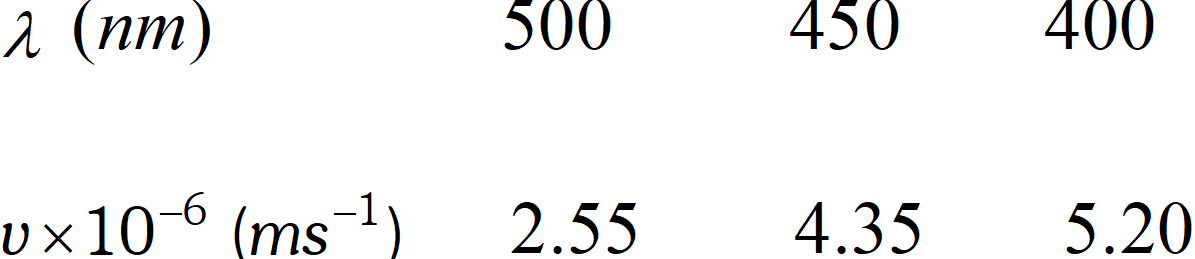[NCERT]
Ans. Suppose threshold wavelength $=\lambda_{0} n m=\lambda_{0} \times 10^{-9} \mathrm{m}$ Then $h\left(v-v_{0}\right)=\frac{1}{2} m v^{2} \quad$ or $\quad h c\left(\frac{1}{\lambda}-\frac{1}{\lambda_{0}}\right)=\frac{1}{2} m v^{2}$ Substituting the given results of the three experiments, we get $\frac{h c}{10^{-9}}\left(\frac{1}{500}-\frac{1}{\lambda_{0}}\right)=\frac{1}{2} m\left(2.55 \times 10^{6}\right)^{2}$ $\frac{h c}{10^{-9}}\left(\frac{1}{450}-\frac{1}{\lambda_{0}}\right)=\frac{1}{2} m\left(4.35 \times 10^{6}\right)^{2}$ $\frac{h c}{10^{-9}}\left(\frac{1}{400}-\frac{1}{\lambda_{0}}\right)=\frac{1}{2} m\left(5.20 \times 10^{6}\right)^{2}$ Dividing equation (ii) by equation (i), we get $\frac{\lambda_{0}-450}{450 \lambda_{0}} \times \frac{500 \lambda_{0}}{\lambda-500}=\left(\frac{4.35}{2.55}\right)^{2}$ or $\quad \frac{\lambda_{0}-450}{\lambda_{0}-500}=\frac{450}{500}\left(\frac{4.35}{2.55}\right)^{2}=2.619$ or $\lambda_{0}-450=2.619 \lambda-1309.5$ or $\quad 1.619 \lambda_{0}=859.5 \quad \therefore \quad \lambda_{0}=531 \mathrm{nm}$ Substituting this value in equation (iii), we get $\frac{h \times\left(3 \times 10^{8}\right)}{10^{-9}}\left(\frac{1}{400}-\frac{1}{531}\right)=\frac{1}{2}\left(9.11 \times 10^{-31}\right)\left(5.20 \times 10^{6}\right)^{2}$ or $\quad h=6.66 \times 10^{-34} \mathrm{Js}$
Q. For which hydrogen like ion the wavelength difference between the first lines of the Lyman and Balmer series is equal to 59.3 nm
Ans. For a spectral line, $\frac{1}{\lambda}=R Z^{2}\left[\frac{1}{n_{1}^{2}}-\frac{1}{n_{2}^{2}}\right]$ For Lyman line, $\frac{1}{\lambda_{\mathrm{Lyman}}}=R Z^{2}\left[\frac{1}{1^{2}}-\frac{1}{2^{2}}\right]=\frac{3 R Z^{2}}{4}$ or $\quad \lambda_{L y \operatorname{man}}=\frac{4}{3 R Z^{2}}$ For Balmer line, $\frac{1}{\lambda_{\text {Balmer }}}=R z^{2}\left[\frac{1}{2^{2}}-\frac{1}{3^{2}}\right]=\frac{5 R Z^{2}}{36}$ or $\quad \lambda_{\text {Balmer }}=\frac{36}{5 R Z^{2}}$ $\lambda_{\text {Balmer }}-\lambda_{\text {Lyman }}=\frac{36}{5 R Z^{2}}-\frac{4}{3 R Z^{2}}=59.3 \times 10^{-7}$ $=\frac{1}{R Z^{2}}\left[\frac{36}{5}-\frac{4}{3}\right]=59.3 \times 10^{-7}$ $=\frac{1}{109677.8 Z^{2}}\left[\frac{108-20}{15}\right]=59.3 \times 10^{-7}$ or $\quad Z^{2}=\frac{1}{109677.8} \times \frac{88}{15 \times 59.3 \times 10^{-7}}=9$ or $\quad Z=3 .$ This corresponds to $L i^{2+}$ ion.
Q. Calculate the velocity $\left(\mathrm{cms}^{-1}\right)$ of an electron placed in third orbit of the hydrogen atom. Also calculate the number of revolutions per second that this electron makes around the nucleus.
Ans. Velocity of electron is given as : $v=\frac{2 \pi e^{2}}{n h}$ Now, $e=4.8 \times 10^{-10}$ e.s.u., $n=3, h=6.63 \times 10^{-27}$ erg sec $\therefore \quad v=\frac{2 \times 22 \times\left(4.8 \times 10^{-10}\right)^{2}}{7 \times 3 \times 6.63 \times 10^{-27}}=7.27 \times 10^{7} \mathrm{cm} \mathrm{s}^{-1}$ No. of revolutions per second $=\frac{\text { Velocity }}{\text { Circumference }}=\frac{v}{2 \pi r}$ but $r=\frac{n^{2} h^{2}}{4 \pi^{2} m e^{2}}$ $\therefore$ No. of revolutions $=\frac{2 \pi e^{2}}{n h} \times \frac{4 \pi^{2} m e^{2}}{2 \pi n^{2} h^{2}}=\frac{4 \pi^{2} m e^{4}}{n^{3} h^{3}}$ $=\frac{4 \times\left(\frac{22}{7}\right)^{2} \times\left(9.1 \times 10^{-28}\right) \times\left(4.8 \times 10^{-10}\right)^{4}}{3^{3} \times\left(6.62 \times 10^{-27}\right)^{3}}$ $=2.42 \times 10^{14}$
Q. Calculate the wavelength in Angstroms of the photon that is emitted when an electron in Bohr orbit $n=2$ returns to the orbit $n=1$ in the hydrogen atom. The ionisation potential of the ground state of hydrogen atom is $2.17 \times 10^{-11}$ ergs per atom.
Ans.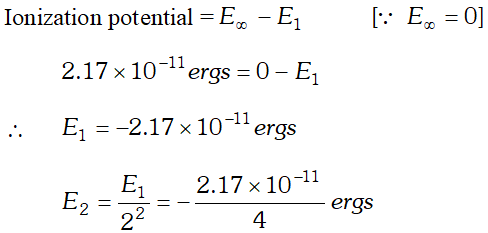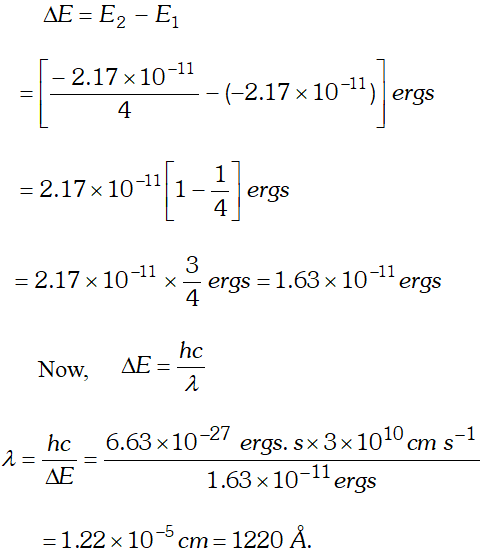Q. The angular momentum of an electron in Bohr's orbit of hydrogen atom is $4.22 \times 10^{-34} \mathrm{kg} \mathrm{m}^{2} \mathrm{s}^{-1} .$ Calculate the wavelength of the spectral line when the electron falls from this level to the next lower level.
Ans. Angular momentum $(m v r)=n \frac{h}{2 \pi}$ $=4.22 \times 10^{-34} \mathrm{kg} \mathrm{m}^{2} \mathrm{s}^{-1}$ (Given) $\therefore \quad n=4.22 \times 10^{-34} \times \frac{2 \pi}{h}$ $=\frac{2 \times 4.22 \times 10^{-34} \times 3.14}{6.626 \times 10^{-34}}=4$ When the electron jumps from $n=4$ to $n=3,$ the wavelength of the spectral line can be calculated as follows: $\frac{1}{\lambda}=R_{H}\left(\frac{1}{n_{1}^{2}}-\frac{1}{n_{2}^{2}}\right)$ $=109,677 \mathrm{cm}^{-1}\left(\frac{1}{3^{2}}-\frac{1}{4^{2}}\right)$ $=109,677 \times\left(\frac{1}{9}-\frac{1}{16}\right)=109,677 \times \frac{7}{144} \mathrm{cm}^{-1}$ or $\lambda=\frac{144}{109,677 \times 7} \mathrm{cm}=1.88 \times 10^{-4} \mathrm{cm}$
Q. (i) Write the electronic configuration of $C u^{+}$ ion $(Z=29). (ii) Calculate the de-Broglie wavelength of a milligram sized object moving with$1 \%$speed of light. Planck's constant$(h)=6.63 \times 10^{-34} \mathrm{kg} \mathrm{m}^{2} \mathrm{s}^{-1}$Velocity of light$(c)=3.0 \times 10^{8} \mathrm{ms}^{-1}$Ans. (i)$\quad C u^{+}(29) 1 s^{2} 2 s^{2} 2 p^{6} 3 s^{2} 3 p^{6} 4 s^{0} 3 d^{10}$(ii)$\quad m=1 m g \quad v=1 \% \times 3 \times 10^{8} m s^{-1}m=10^{-6} \mathrm{kg} \quad v=\frac{1}{100} \times 3 \times 10^{8} \mathrm{ms}^{-1}=3 \times 10^{6} \mathrm{ms}^{-1}\lambda=\frac{h}{m v}=\frac{6.63 \times 10^{-34} \mathrm{kg} m^{2} s^{-1}}{10^{-6} \mathrm{kg} \times 3 \times 10^{6} \mathrm{ms}^{-1}}=2.21 \times 10^{-34} \mathrm{m}$Q. Give the electronic configuration of the following ions: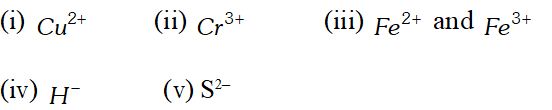Ans. During the formation of cations, electrons are lost while in the formation of anions, electrons are added to the valence shell. The number of electrons added or lost is equal to the numerical value of the charge present on the ion. Following this general concept, we can write the electronic configurations of all the ions given in the question. (i)$\quad C u^{2+}=_{29} C u-2 e^{-}=1 s^{2} 2 s^{2} 2 p^{6} 3 s^{2} 3 p^{6} 3 d^{10} 4 s^{1}-2 e^{-}=1 s^{2} 2 s^{2} 2 p^{6} 3 s^{2} 3 p^{6} 3 d^{9}$(ii)$\quad C r^{3+}=_{24} C r-3 e^{-}=1 s^{2} 2 s^{2} 2 p^{6} 3 s^{2} 3 p^{6} 3 d^{5} 4 s^{1}-3 e^{-}=1 s^{2} 2 s^{2} 2 p^{6} 3 s^{2} 3 p^{6} 3 d^{3}$(iii)$\quad F e^{2+}=_{26} F e-2 e^{-}=1 s^{2} 2 s^{2} 2 p^{6} 3 s^{2} 3 p^{6} 3 d^{6} 4 s^{2}-2 e^{-}=1 s^{2} 2 s^{2} 2 p^{6} 3 s^{2} 3 p^{6} 3 d^{6}=1 s^{2} 2 s^{2} 2 p^{6} 3 s^{2} 3 p^{6} 3 d^{6}F e^{3+}=26 F e-3 e^{-}=1 s^{2} 2 s^{2} 2 p^{6} 3 s^{2} 3 p^{6} 3 d^{5}$(iv)$\quad H^{-}=_{1} H+1 e^{-}=1 s^{1}+1 e^{1}=1 s^{2}$(v)$\quad S^{2-}=_{16} S+2 e^{-}=1 s^{2} 2 s^{2} 2 p^{6} 3 s^{2} 3 p_{x}^{2} 3 p_{y}^{1} 3 p_{z}^{1}+2 e^{-}=1 s^{2} 2 s^{2} 2 p^{6} 3 s^{2} 3 p_{x}^{2} 3 p_{y}^{2} 3 p_{z}^{2}\$

Deborah
Sept. 7, 2021, 2:23 p.m.
Questions are very nice but why they have no solutions
L
June 1, 2021, 1:48 p.m.
Saman golo see bani ek sanrachna mein core lambai 0.8 millimeter hai . Parmado ki trijya gyat Karo yadi yaha b.c.c. jalak hai
Shiva
Jan. 28, 2021, 4:57 p.m.
Structure of atoms important question ❓
Asharam
Jan. 21, 2021, 9:09 a.m.
I want still more important than this all please send to 9381438347 number I like this type of questions
Dec. 15, 2020, 9:14 a.m.
Worst website that I have ever seen you have to really improve it because there are so many mistakes in answer not Notsatisfied..........................
CRACK NEET
Dec. 7, 2020, 12:49 p.m.
This website along with <a href="allen4all.blogspot.com" rel="nofollow ugc">allen4all</a> help me lot
Dec. 7, 2020, 12:46 p.m.
This website along with <a href="allen4all.blogspot.com" rel="nofollow ugc">allen4all.</a> help me lot.
Aruna
Nov. 7, 2020, 2:46 p.m.
Mrinal
Oct. 9, 2020, 1:15 p.m.
I see u i say o shit
Abhishek
Oct. 9, 2020, 1:14 p.m.
Follow @silverwatermelon for thick water and melons
Burhan
Oct. 9, 2020, 1:13 p.m.
Burhan is lame
Ishita paul
Oct. 7, 2020, 8:47 p.m.
Really Very good and also very helpful for practice